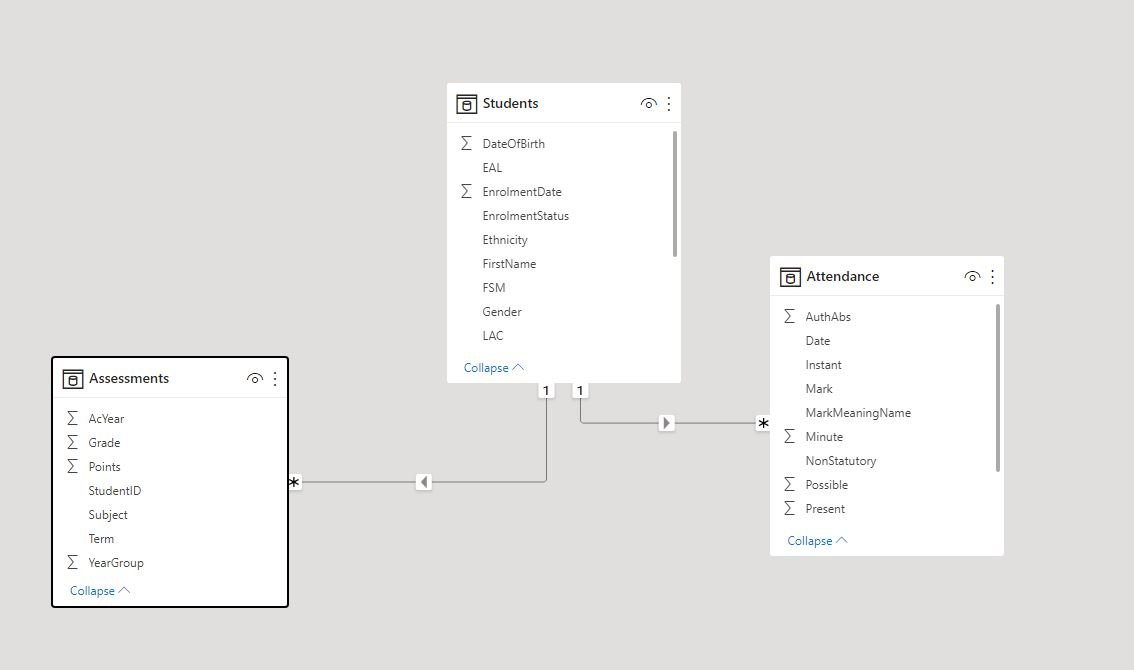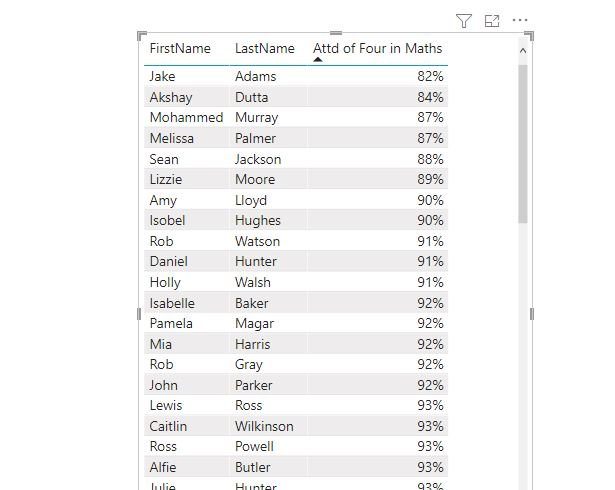# Filtering with multiple fact tables

If you read BI best practice guides they’ll tell you to build a data model in star schema, which is where you the record of what’s happened in a big table called a fact table and records of who it happened to, where etc in smaller ‘Dimension’ tables. The primary keys of the dimension table link to foreign keys on the fact table so they look like points of a star, hence ‘star schema’.

However, in a school setting we typically have multiple ‘fact’ records like attendances, behaviour incidents and assessment results that can’t be easily combined into one big table. Plus we often want to report across these areas – find the attendance of students who are below target for example.

There are a couple of ways we can do this, using FILTER and TREATAS, and which one is best will depend on whether you can leverage relationships (usually preferable) or not.

### Set Up

Imagine I have an assessments table where each record has StudentID, subject and grade. I’ve got an attendance table too with columns containing 1s/0s for Present and Possible and both of these tables are linked to a students table with one to many relationships.To keep things simple I’ll refer to a couple of simple measures, [APS] (Average Points Score) as the average assessment and [Attendance] where

```            ```
APS = AVERAGE(Assessment[Points])
Attendance = DIVIDE(
SUM(Attendance[Present]),
SUM(Attendance[Possible])
)
```
```

### Attainment of Persistent Absentees

Let’s say we want to know the average points score of persistent absentees. What we’re going to do is work out the ids of all students whose attendance is 90% or lower, then filter our assessment results to just those students.

You can do the first part with FILTER(Students,[Attendance] <= 0.9) then use that filter on the students table as a calculate modifier. So your final measure looks like

```            ```
APS of PA =
CALCULATE([APS],
FILTER(Students,[Attendance]<=.9))
```
```

Note that this works because FILTER is an iterator which works through the students table working out the attendance of each row/child. Just putting [Attendance] <= .9 as a calculate filter would throw an error.

### Filtering without relationships

This technique works because both attendance and assessments are filtered by students. But say we want to know the attainment of students who are taught by NQTs. There isn’t an obvious way to connect staff and class information to our assessments table with a relationship.

What we can do instead is get a table of values from one table and use it to filter values in another table via the TREATAS function.

```            ```
APS of NQT =
VAR NQTStudentSubCombos = CALCULATETABLE(
SUMMARIZE(Classes,Classes[StudentID],Classes[Subject]),
Staff[NQT]="Y")
VAR R = CALCULATE([APS],
TREATAS(NQTStudentSubCombos,Assessments[StudentID],Assessments[Subject]))
RETURN
R
```
```

The variable NQTStudentSubCombos gets a list of every student id / subject combination where the teacher is an NQT from the Classes table. The TREATAS calculate modifier then filters the assessments table to rows which have these combinations.

Note that the order of the columns: student ID then subject needs to be the same in the SUMMARIZE and TREATAS functions. Note also that the subjects need to be identical – you may get tripped up, for example, if your class subject is English but your Assessment Subject is English Language. I recommend resolving such issues when data is imported using Power Query.

### Attendance based on Assessment

TREATAS does not need to match on two columns – in fact a more common use case would be for translating a single identifier between fact tables. I use the formulation CALCULATETABLE(VALUES(… for this.

For example, to list students getting a 4 in maths with their attendance you could create the measure:

```            ```
Attd of Four in Maths =
VAR FinM = CALCULATETABLE(
VALUES(Assessments[StudentID]),
Assessments[Subject] = "Mathematics",
Assessments[Points] = 4)
RETURN
CALCULATE([Attendance],
TREATAS(FinM,Attendance[StudentID]))
```
```

As this measure will only return a result for students with a four in maths then placing it into a table with student names automatically filters to just those students.